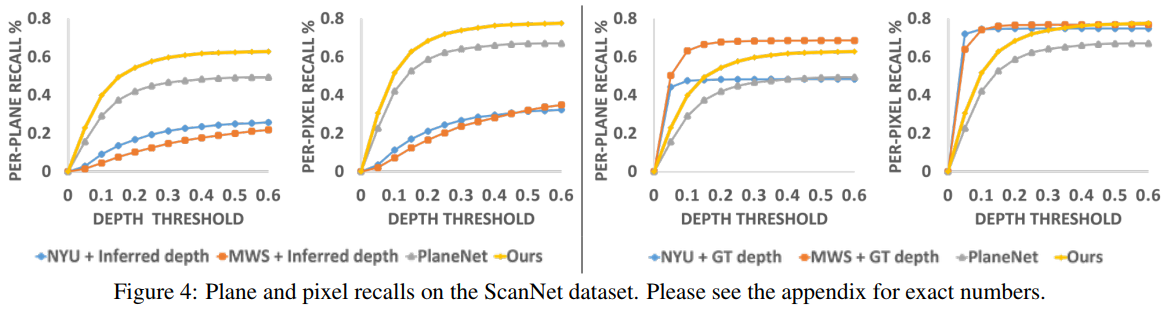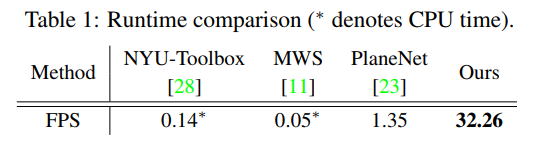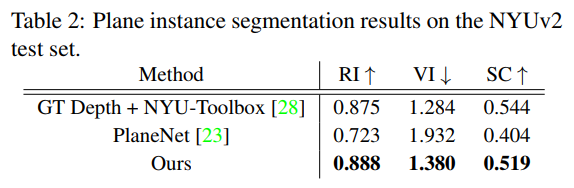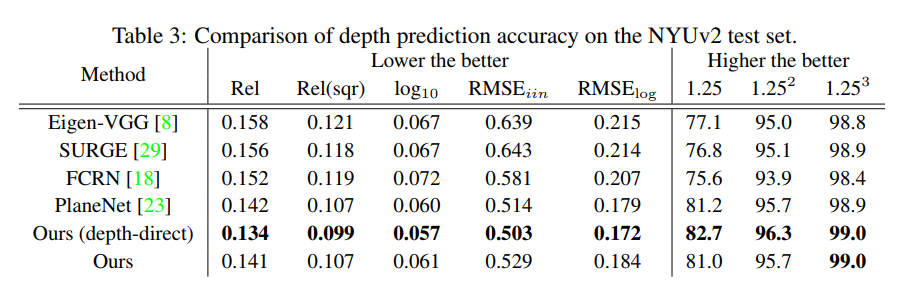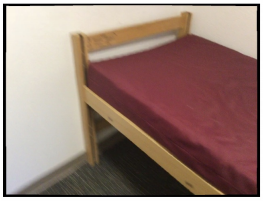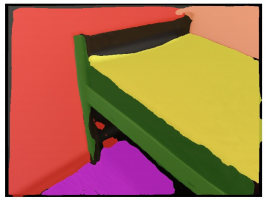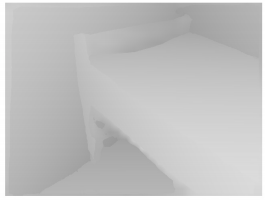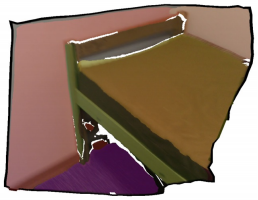input image piece-wise planar segmentation reconstructed depthmap texture-mapped 3D model

## 0x00 Datasets

• ScanNet [1,3,4]
• SYNTHIA [2,3]
• Cityscapes 
• NYU Depth Dataset [1,3,4]
• Labeling method

ScanNet: Richly-annotated 3D Reconstructions of Indoor Scenes. annotated with 3D camera poses, surface reconstructions, and instance-level semantic segmentations.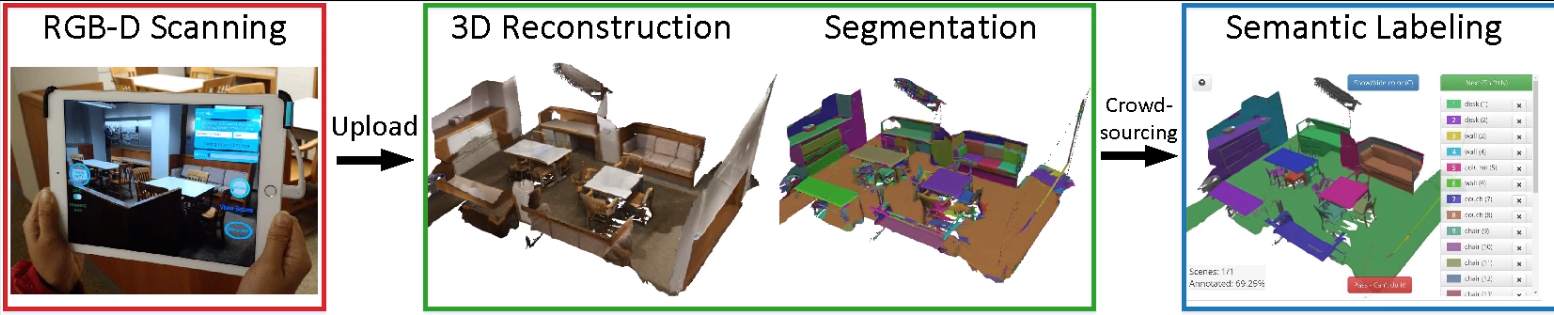SYNTHIA: The SYNTHetic collection of Imagery and Annotations. 8 RGB cameras forming a binocular 360º camera, 8 depth sensors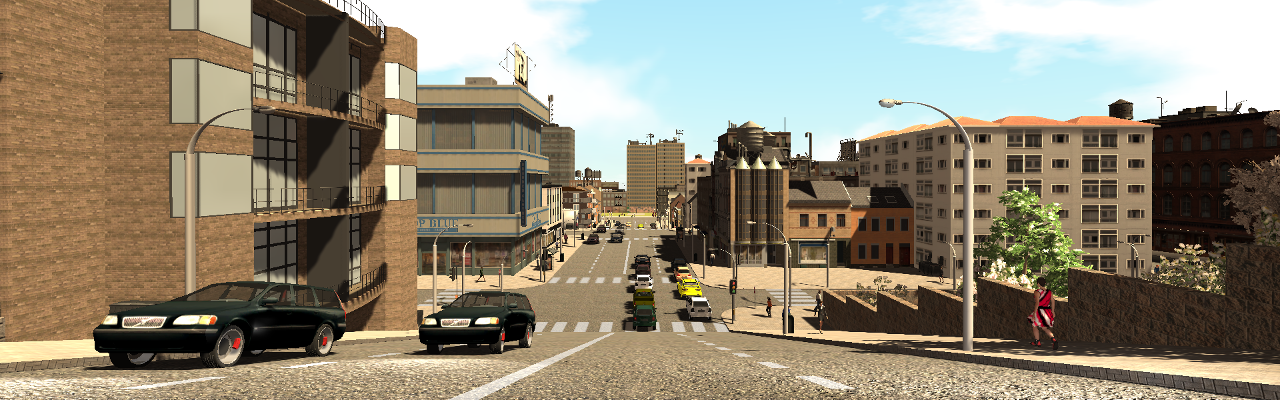Cityscapes: Benchmark suite and evaluation server for pixel-level and instance-level semantic labeling.
video frames / stereo / GPS / vehicle odometry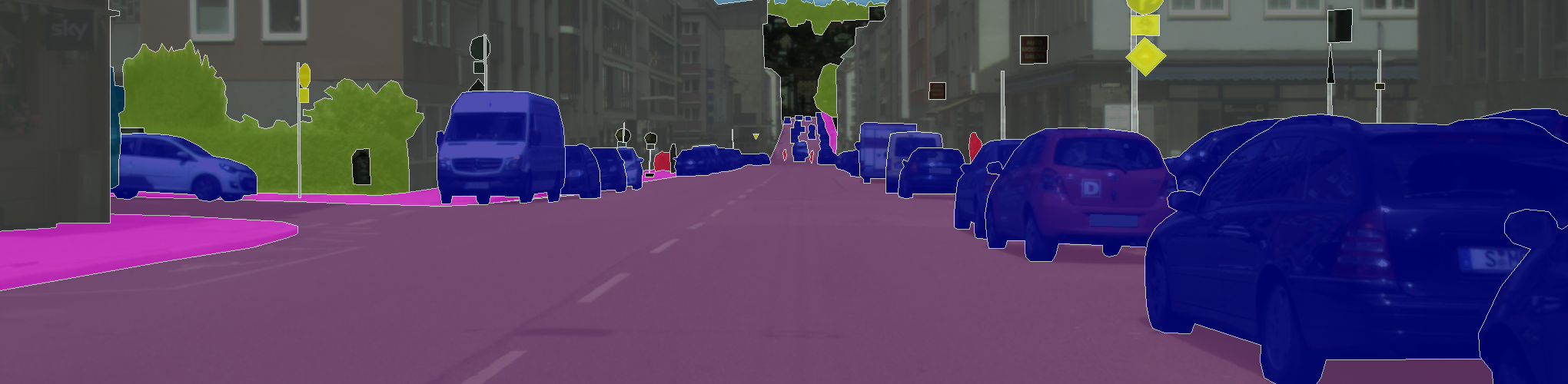NYU Depth Dataset: is recorded by both the RGB and Depth cameras from the Microsoft Kinect.

• Dense multi-class labels with instance number (cup1, cup2, cup3, etc).
• Raw: The raw rgb, depth and accelerometer data as provided by the Kinect.
• Toolbox: Useful functions for manipulating the data and labels.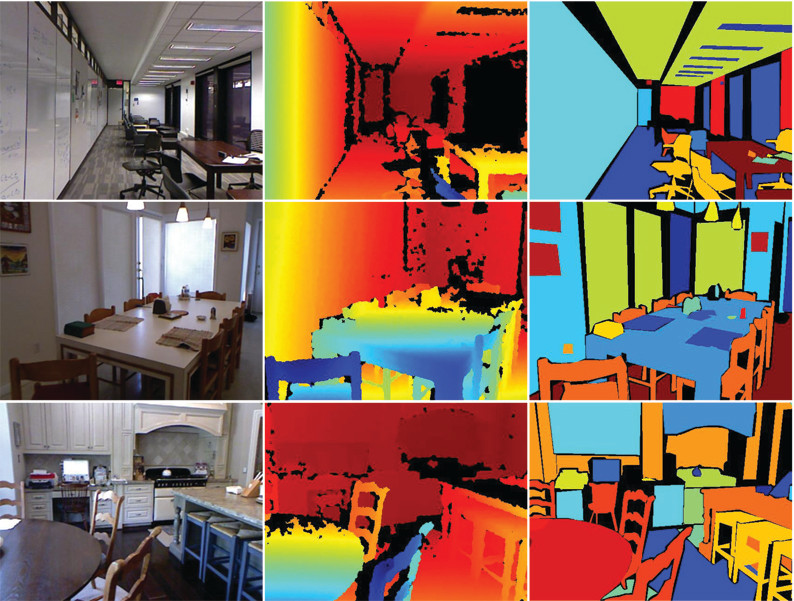### Obtaining ground truth plane annotations :

Difficulty in detect planes from the 3D point cloud by using J-Linkage method.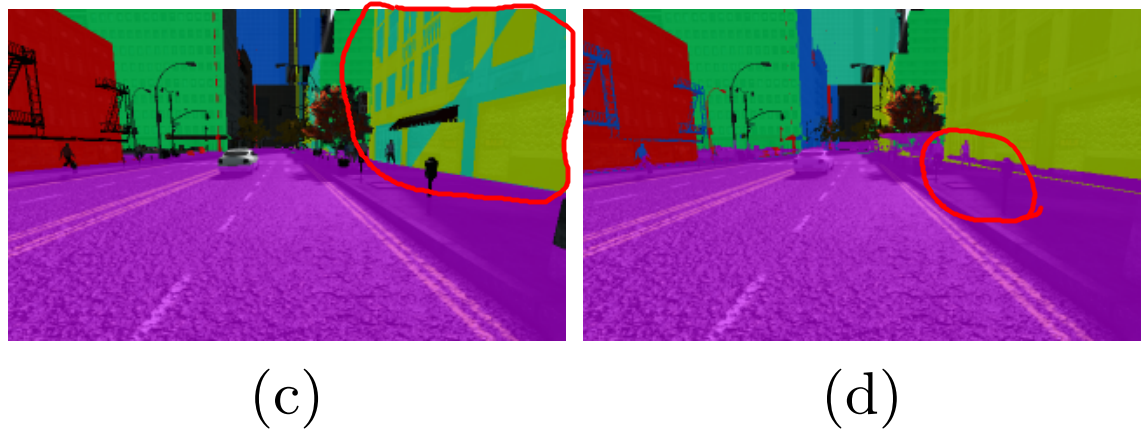(c-d): Plane fitting results generated by J-Linkage with δ = 0.5 and δ = 2, respectively.

### Labeling method:

ScanNet:
1. Fit plans to a consolidated mesh (merge planes if (normal diff < 20° && distance < 5cm)
2. Project plans back to individual frames
SYNTHIA:
1. Manually draw a quadrilateral region
2. Obtain the plane parameters and variance of the distance distribution
3. Find all pixels that belong to the plane by using the plane parameters and the variance estimate
Cityscapes:
1. “planar” = {ground, road, sidewalk,parking, rail track, building, wall, fence, guard rail, bridge, and terrain}
2. Manually label the boundary of each plane using polygons

## 0x01 PlaneNet

[CVPR 2018] Liu, Chen, et al. Washington University in St. Louis, Adobe.

The first deep neural architecture for piece-wise planar depthmap reconstruction from a RGB image.

### Pipeline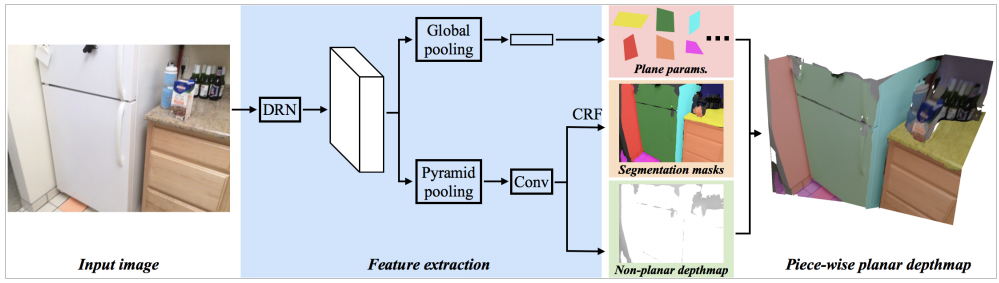DRN: Dilated Residual Networks (2096 channels)

CRF: Conditional Random Field Algorithm

Step Loss
Plane parameter: $$L^P=\sum_{i=1}^{K^*}min_{j\in[1,K]}\Vert P_i^*-P_j \Vert_2^2 \;\;\; (K = 10)$$
Plane segmentation: softmax cross entropy $$L^M=\sum_{i=1}^{K+1}\sum_{p \in I}(1(M^{*(p)}=i)log(1-M_i^{(p)}))$$
Non-planar depth: ground-truth <==> predicted depthmap $$L^D=\sum_{i=1}^{K+1}\sum_{p\in I}(M_i^{(p)}(D_i^{(p)}-D^{*(p)})^2)$$
- $M^{(p)}\text{: probability of p belonging to the } i^{th} \text{ plane ;}\\ D^{(p)} \text{: depth value at pixel }p \text{ ;}\\ \text{*: GT .}$

## 0x02 Plane Recover

[ECCV 18] Fengting Yang and Zihan Zhou Pennsylvania State University.

Recovering 3D Planes from a Single Image. Propose a novel plane structure-induced loss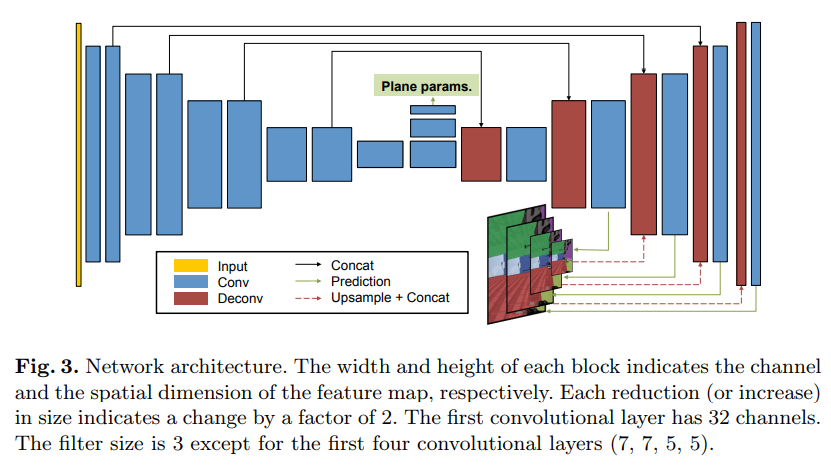Step Loss
Plane loss $$L_{reg}(S_{i})=\sum_{q}^{}-z(q)\cdot log(p_{plane}(q))-(1-z(q))\cdot log(1-p_{plane}(q))$$
Loss $$L=\sum_{i=1}^{n}\sum_{j=1}^m\left(\sum_{q}S_{i}^{j}(q)\cdot \vert(n_{i}^{j})^{T}Q-1\vert\right)+\alpha \sum_{i=1}^{n}L_{reg}(S_{i})$$

## 0x03 PlaneRCNN

[CVPR2019] Liu, Chen, et al. NVIDIA, Washington University in St. Louis, SenseTime, Simon Fraser University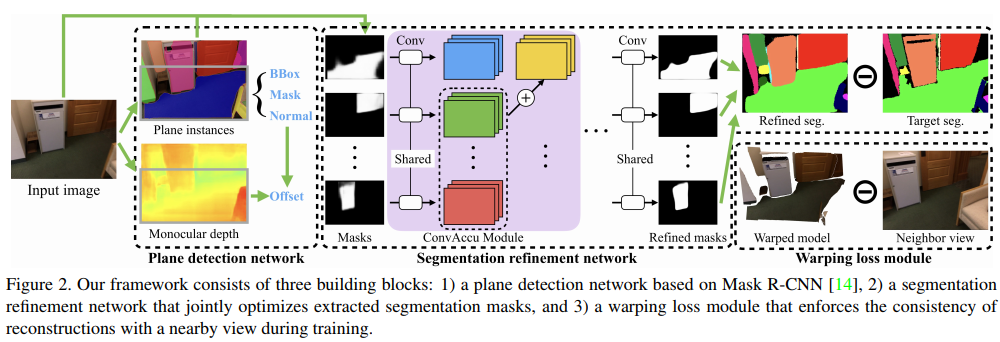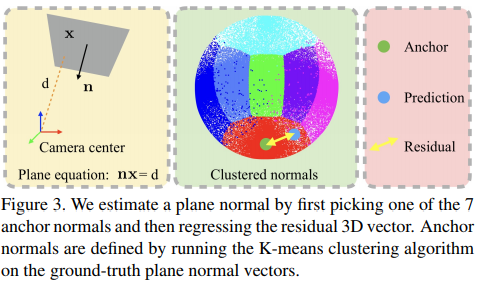## 0x04 PlanarReconstruction

[CVPR 2019] Yu, Zehao, et al. ShanghaiTech University, The Pennsylvania State University

Single-Image Piece-wise Planar 3D Reconstruction via Associative Embedding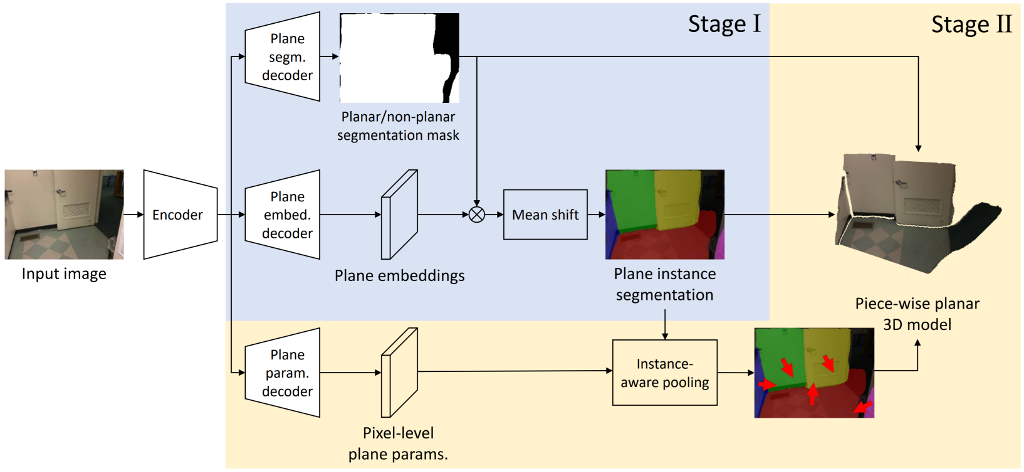Step Loss
Segmentation: balanced cross entropy $$L_{S}=-(1-w)\sum_{i\in\mathcal{F}}^{}\log p_{i}-w\sum_{i\in\mathcal{B}}^{}\log(1-p_{i})$$
Embedding: discuiminative loss $$L_{E}=L_{pull}+L_{push}$$
Per-pixel plane: L1 loss $$L_{PP}=\frac{1}{N}\sum_{i=1}^{N}\vert n_{i}-n^{*}_{i}\ \vert$$
Instance Parameter: $$L_{IP}=\frac{1}{N\tilde{C}}\sum_{j=1}^{\tilde{C}}\sum_{i=1}^{N}S_{ij}\cdot\vert n_{j}^{T}Q_{i}-1\vert$$
Loss $$L=L_{S}+L_{E}+L_{PP}+L_{IP}+…$$

Embedding:
associative emvedding (End-to-End Learning for Joint Detection and Grouping) ;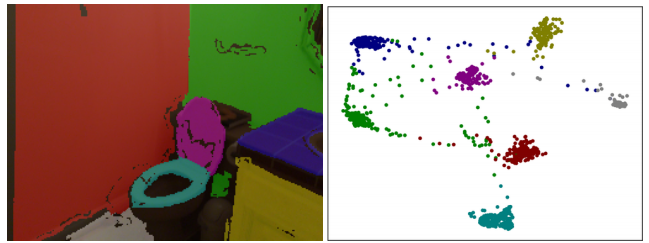### Discriminative loss function

• An image can contain an arbitrary number of instances
• The labeling is permutation-invariant: it does not matter which specific label an instance gets, as long as it is different from all otherinstance labels.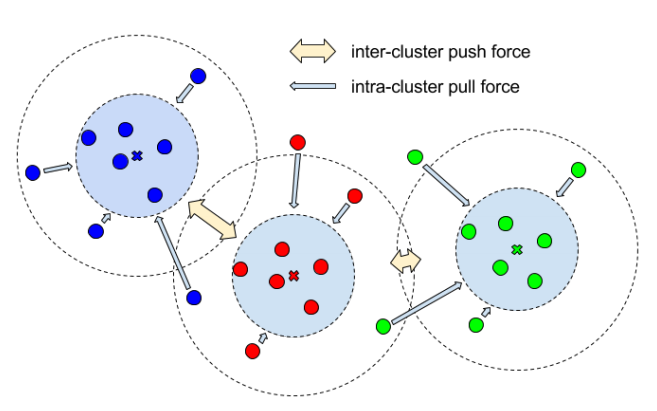$$L_{E}=L_{pull}+L_{push}$$

$$where$$

$$L_{pull}=\frac{1}{C}\sum_{c=1}^{C}\frac{1}{N_{c}}\sum_{i=1}^{N_{c}}\max\left(\lVert\mu_{c}-x_{i}\rVert-\delta_{\textrm{v}},0\right)$$

$$L_{push}=\frac{1}{C(C-1)}\mathop{\sum_{c_{A}=1}^{C}\sum_{c_{B}=1}^{C}}_{c_{A}\neq c_{B}}\max\left(\delta_{\textrm{d}}-\lVert\mu_{c_{A}}-\mu_{c_{B}}\rVert,0\right)$$

Here, $C$ is the number of clusters $C$ (planes) in the ground truth, $N_c$ is the number of elements in cluster $c$, $x_i$ is the pixel embedding, $μ_c$ is the mean embedding of the cluster $c$, and $δ_v$ and $δ_d$ are the margin for “pull” and “push” losses, respectively.

Instance Parameter Loss:

$$L_{IP}=\frac{1}{N\tilde{C}}\sum_{j=1}^{\tilde{C}}\sum_{i=1}^{N}S_{ij}\cdot\vert n_{j}^{T}Q_{i}-1\vert$$ $S\text{: instance segmentation map}\\n_{j}\text{: predicted plane param}\\Q_i\text{: the 3D point at pixel } i$

$n\doteq\tilde{n}/d$ , where $\tilde{n}\in\mathcal{S}^{2}$ and $d$ denote the surface normal and plane distance to the origin

## 0xFF Results

### PlaneNet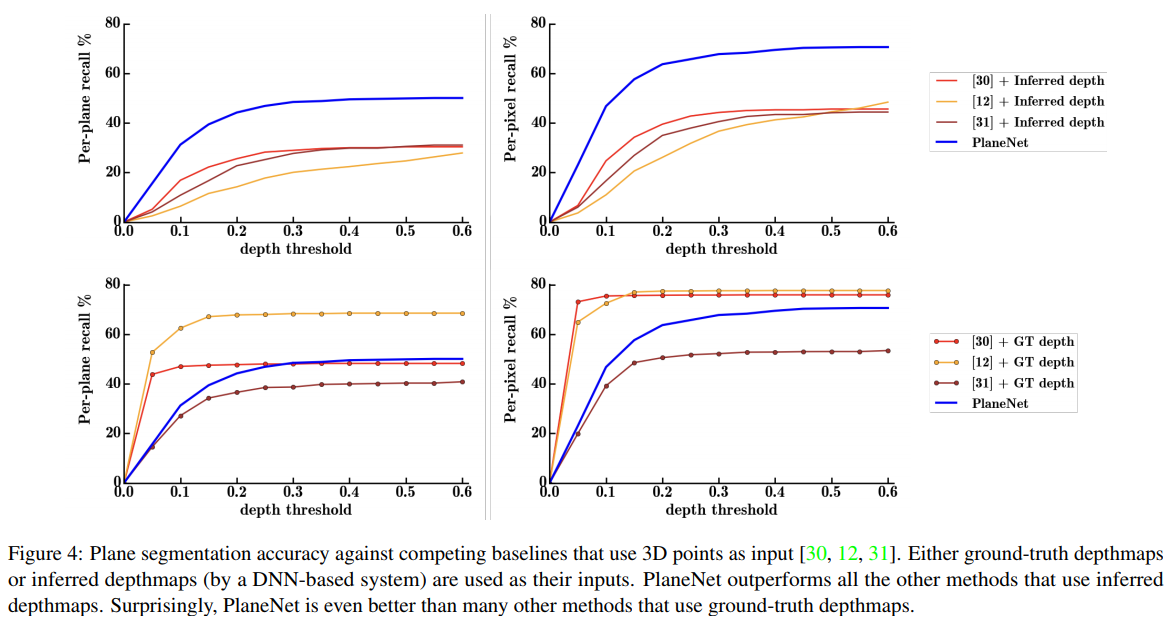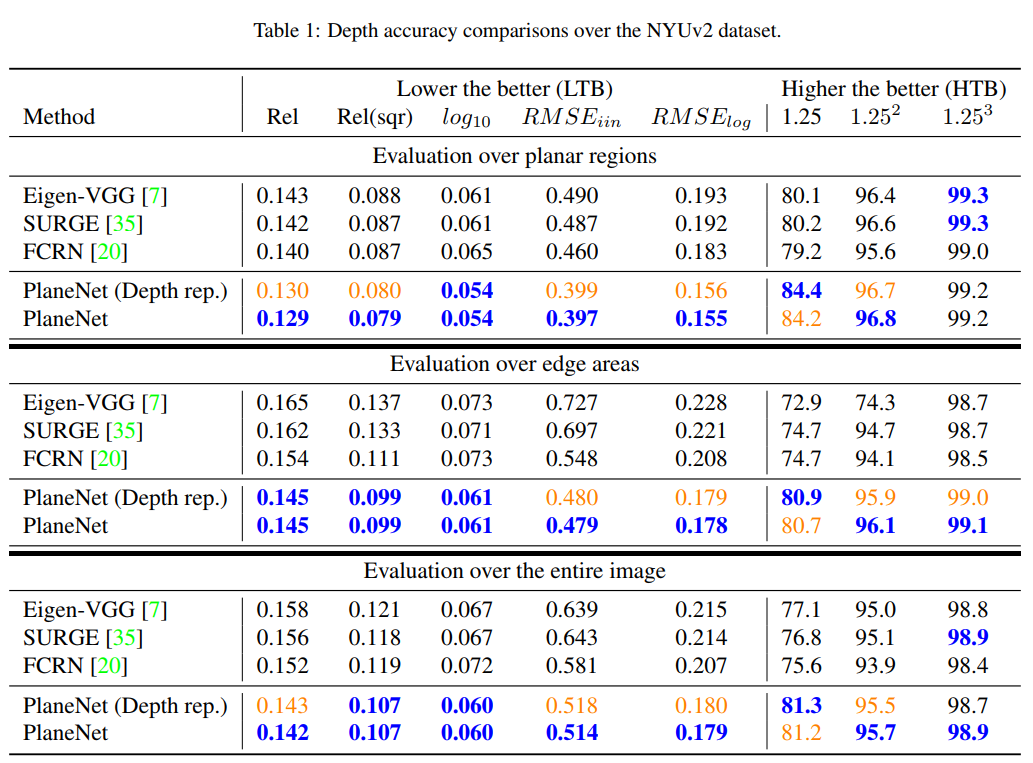### PlaneRecover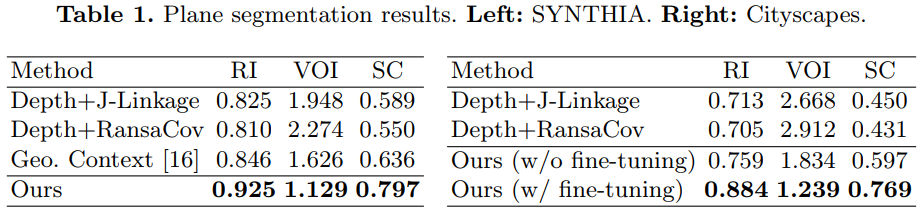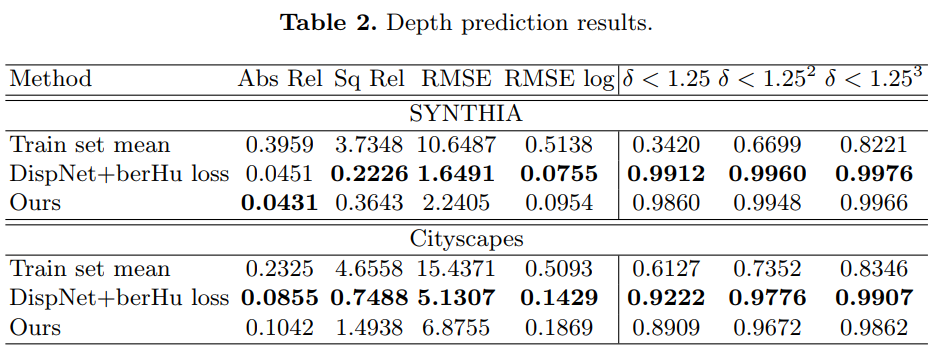### PlaneRCNN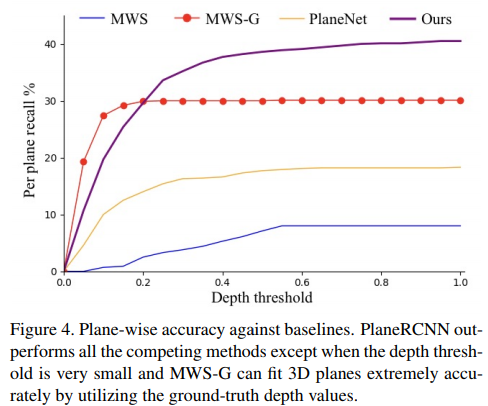### PlanarReconstruction